# PSAT Math : How to find out if lines are perpendicular

## Example Questions

### Example Question #1 : Perpendicular Lines

Which set of lines is perpendicular?

y = 3x/5 – 3 and y = 5x/3 + 3

the line between the points (7,4) and (4,7), and the line between the points (3,9) and (4,8)

y = 3x + 5 and y = 5x + 3

y = x – 1/2 and y = –x + 1/2

the line between the points (1,3) and (3,5), and y = 4x + 7

y = x – 1/2 and y = –x + 1/2

Explanation:

Two lines are perpendicular to each other if their slopes are negative reciprocals. For example, if one line has a slope of 2, the line perpendicular to it has a slope of –1/2. One easy way to eliminate answer choices is to check if the slopes have the same sign, i.e. both positive or both negative. If so, they cannot be perpendicular. Several of the lines in the answer choices are of the form y = mx + b, where m is the slope and b is the y-intercept. We are only worried about the slope for the purposes of this question.

y = 3x + 5 and y = 5x + 3 both have positive slopes (m = 3 and m = 5, respectively), so they aren't perpendicular.

y = 3x/5 – 3 and y = 5x/3 + 3 both have positive slopes, so again they aren't perpendicular.

y = x – 1/2 and y = –x + 1/2 have slopes of m = 1 and m = –1, respectively. One is positive and one is negative, so that is a good sign. Let's take the negative reciprocal of 1. 1 /–1 = –1. So these two slopes are in fact negative reciprocals, and these two lines are perpendicular to each other. Even though we have found the correct answer, let's go through the other two choices to be sure.

The line between the points (1,3) and (3,5), and y = 4x + 7: We need to find the slope of the first line. slope = rise / run = (y2 – y1) / (x2 – x1) = (5 – 3) / (3 – 1) = 1. The slope of y = 4x + 7 is also positive (m = 4), so the lines are not perpendicular.

The line between the points (7,4) and (4,7), and the line between the points (3,9) and (4,8): the first slope = (7 – 4) / (4 – 7) = –1 and the second slope = (8 – 9) / (4 – 3) = –1. They have the same slope, making them parallel, not perpendicular.

### Example Question #1 : How To Find Out If Lines Are Perpendicular

Which set of lines is perpendicular?

y = 3x + 5 and y = 5x + 3

the line between the points (1,3) and (3,5), and y = 4x + 7

y = 3x/5 – 3 and y = 5x/3 + 3

y = x – 1/2 and y = –x + 1/2

the line between the points (7,4) and (4,7), and the line between the points (3,9) and (4,8)

y = x – 1/2 and y = –x + 1/2

Explanation:

Two lines are perpendicular to each other if their slopes are negative reciprocals. For example, if one line has a slope of 2, the line perpendicular to it has a slope of –1/2. One easy way to eliminate answer choices is to check if the slopes have the same sign, i.e. both positive or both negative. If so, they cannot be perpendicular. Several of the lines in the answer choices are of the form y = mx + b, where m is the slope and b is the y-intercept. We are only worried about the slope for the purposes of this question.

y = 3x + 5 and y = 5x + 3 both have positive slopes (m = 3 and m = 5, respectively), so they aren't perpendicular.

y = 3x/5 – 3 and y = 5x/3 + 3 both have positive slopes, so again they aren't perpendicular.

y = x – 1/2 and y = –x + 1/2 have slopes of m = 1 and m = –1, respectively. One is positive and one is negative, so that is a good sign. Let's take the negative reciprocal of 1. 1 /–1 = –1. So these two slopes are in fact negative reciprocals, and these two lines are perpendicular to each other. Even though we have found the correct answer, let's go through the other two choices to be sure.

The line between the points (1,3) and (3,5), and y = 4x + 7: We need to find the slope of the first line. slope = rise / run = (y2 – y1) / (x2 – x1) = (5 – 3) / (3 – 1) = 1. The slope of y = 4x + 7 is also positive (m = 4), so the lines are not perpendicular.

The line between the points (7,4) and (4,7), and the line between the points (3,9) and (4,8): the first slope = (7 – 4) / (4 – 7) = –1 and the second slope = (8 – 9) / (4 – 3) = –1. They have the same slope, making them parallel, not perpendicular.

### Example Question #471 : Geometry

Which of the following lines is perpendicular to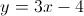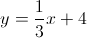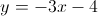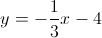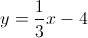Explanation:

The line which is perpendicular has a slope which is the negative inverse of the slope of the original line.

### Example Question #1 : How To Find Out If Lines Are Perpendicular

If two lines have slopes of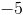and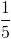, which statement about the lines is true?

They intersect at two points.

They are perpendicular.

They are parabolas.

They are parallel.

They don't intersect.

They are perpendicular.

Explanation:

Perpendicular lines have slopes that are the negative reciprocals of each other.

### Example Question #2 : Perpendicular Lines

Lineincludes the points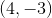and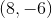. Lineincludes the points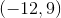and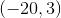. Which of the following statements is true of these lines?

The lines are distinct but neither parallel nor perpendicular.

The lines are perpendicular.

The lines are parallel.

The lines are identical.

The lines are distinct but neither parallel nor perpendicular.

Explanation:

We calculate the slopes of the lines using the slope formula.

The slope of lineis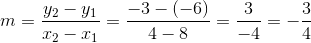The slope of lineis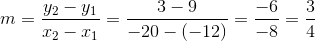Parallel lines and identical lines must have the same slope, so these can be eliminated as choices. The slopes of perpendicular lines must have product. The slopes have product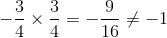so they are not perpendicular.

The correct response is that the lines are distinct but neither parallel nor perpendicular.

### Example Question #1 : How To Find Out If Lines Are Perpendicular

Lineincludes the pointsand. Lineincludes the points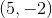and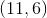. Which of the following statements is true of these lines?

The lines are perpendicular.

The lines are distinct but neither parallel nor perpendicular.

The lines are identical.

The lines are parallel.

Insufficient information is given to answer this question.

The lines are perpendicular.

Explanation:

We calculate the slopes of the lines using the slope formula.

The slope of lineisThe slope of lineis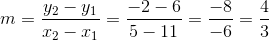The slopes are not the same, so the lines are neither parallel nor identical. We multiply their slopes to test for perpendicularity: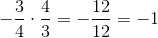The product of the slopes is, making the lines perpendicular.

### Example Question #4 : Perpendicular Lines

Consider the equations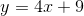and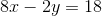. Which of the following statements is true of the lines of these equations?

The lines are perpendicular.

The lines are identical.

The lines are parallel.

The lines are distinct but neither parallel nor perpendicular.

Insufficient information is given to answer this question.

The lines are parallel.

Explanation:

We find the slope of each line by putting each equation in slope-intercept formand examining the coefficient of.is already in slope-intercept form; its slope is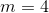.

To getin slope-intercept form we solve for: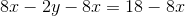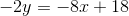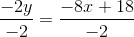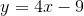The slope of this line is also.

The slopes are equal; however, the-intercepts are different - the-intercept of the first line is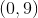and that of the second line is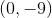. Therefore, the lines are parallel as opposed to being the same line.

### Example Question #51 : Lines

Which line is perpendicular to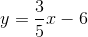?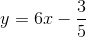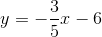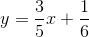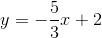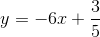To determine if lines are perpendicular, look at the slope. Perpendicular lines have slopes that are negative reciprocals. The slope ofis.  The negative reciprocal ofis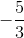.  Therefore, the answer is.